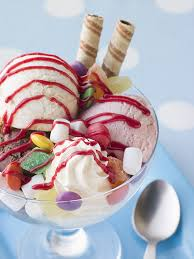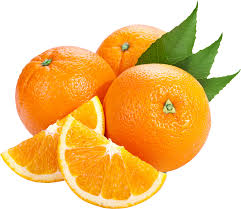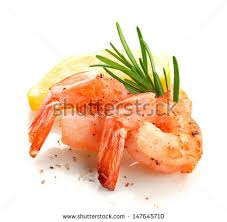# Vivian's website

Welcome to this website!Food I Like
• icecream
•• hamburger
• dumplings
• orange
•Food I Don't Like
• fish
• shrimp
•• yam
• Helping tools
first name:

last name:

first number:

second number:

first number:

second number:

first number:

second number:

snowman background(148,251,255); // RGB //ground: fill(230, 218, 212); rect(10,340,380,50); line(160,190,80,80); line(240,200,340,80); // bottom circle: fill(250, 247, 247); ellipse(200,300,150,150); // middle circle: ellipse(200,200,120,120); // top circle: ellipse(200,110,100,100); ellipse(30,150,13,13); ellipse(280,200,13,13); ellipse(340,300,13,13); ellipse(80,250,13,13); ellipse(280,60,13,13); ellipse(30,360,13,13); ellipse(150,20,13,13); // buttons: fill(247, 255, 0); ellipse(200,190,10,10); ellipse(200,220,10,10); // eyes: ellipse(180,100,20,20); ellipse(220,100,20,20); //hand: ellipse(80,80,15,15); ellipse(340,80,15,15); //pupil: fill(13, 6, 6); ellipse(178,99,10,11); ellipse(218,99,10,11); //nose: fill(255, 140, 0); ellipse(200,120,13,30); //scarf: fill(0, 196, 255); rect(230,150,15,100); rect(157,150,90,15); //hat: rect(170,10,60,60); rect(155,60,90,15); //flower: fill(191, 0, 255); ellipse(220,54,8,15); ellipse(220,66,8,15); ellipse(214,60,15,8); ellipse(226,60,15,8); //center of flower: fill(240, 67, 200); ellipse(220,60,10,10); headband girl //head: fill(247, 255, 0); ellipse(200, 200, 200, 200); //eyes: fill(250, 240, 240); ellipse(150,190,50,50); ellipse(220,190,50,50); //pupil: fill(36, 23, 23); ellipse(212,187,25,25); ellipse(144,187,25,25); //mouth: fill(217, 17, 17); ellipse(190,250,40,60); //face: ellipse(135,230,25,15); ellipse(250,230,35,15); //headband: strokeWeight(25); stroke(214, 39, 202); line(120,140,250,120); line(250,130,280,190); line(260,125,360,210); //badge: fill(101, 81, 214); ellipse(255,130,20,20);